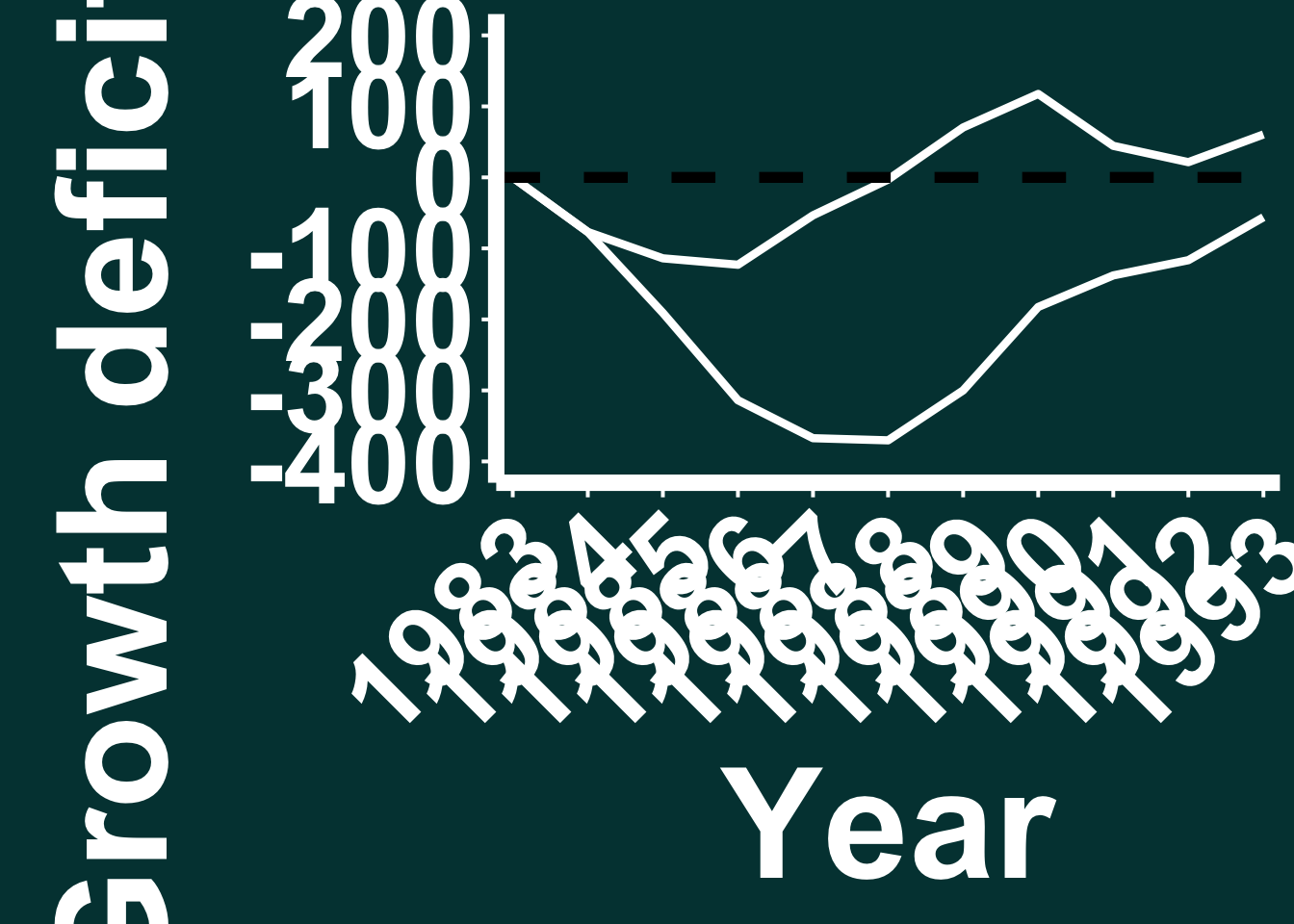Comments: Load in the tidyverse (install it if you don’t already have it) and then set your working directory to where you have saved the downloaded data by changing the below file path. Then, read in the data using the .csv command.

library(tidyverse)
## ── Attaching packages ─────────────────────────────────────── tidyverse 1.3.0 ──
## ✓ ggplot2 3.3.3     ✓ purrr   0.3.4
## ✓ tibble  3.0.6     ✓ dplyr   1.0.2
## ✓ tidyr   1.1.2     ✓ stringr 1.4.0
## ✓ readr   1.4.0     ✓ forcats 0.5.0
## ── Conflicts ────────────────────────────────────────── tidyverse_conflicts() ──
## x dplyr::lag()    masks stats::lag()
setwd("//Users/macbookpro/Creative Cloud Files/YouTube SciComm Tutorial Series/3. Basic animation - animating a graph in After Effects/R_Data/")

AE_Example_data <- read.csv("FR6_BA_BAI_Forecast_vs_Actual.csv")

Comments: Once you have loaded in the ggplot package, set your working directory and loaded in the data using the above steps, we can perform some basic calculations on the data (just summing up different groups of data) and storing them as new dataframes using the pipe command ( %>% ), which is what the next piece of code does.

AE_Example_data %>%
group_by(Stem_height, Year) %>%
summarise(sum = sum(BAI_Annual_Deficit)) -> BAI_Annual_Deficit_sum
## summarise() regrouping output by 'Stem_height' (override with .groups argument)
AE_Example_data %>%
group_by(Stem_height, Year) %>%
summarise(sum = sum(BAI_cumsum_deficit)) -> BAI_cumsum_deficit_sum
## summarise() regrouping output by 'Stem_height' (override with .groups argument)

Comments: Now we can generate a figure from these two new dataset using the ggplot code below (don’t worry that it looks badly formatted in the “plots” panel, we have set the axis and text sizing intentionally so it’s a readable size when we export it. It just looks a mess in the “Plots” panel). Once we have created the ggplot, make sure you save the figure via the “Export” button in the “Plots” panel and save it as an “EPS” NOT AS A “PNG” file. In the tutorial, we de-select the aspect ratio box and save it as 1920 (w) x 1080 (h).

ggplot() +
geom_line(data = BAI_Annual_Deficit_sum, aes(x = Year, y = sum,),  size = 1.5, colour = "white") +
geom_line(data = BAI_cumsum_deficit_sum, aes(x = Year, y = sum,),  size = 1.5, colour = "white") +
scale_x_continuous(expand = c(0.02, 0.02), breaks = c(1983, 1984, 1985, 1986, 1987, 1988, 1989, 1990, 1991, 1992, 1993)) +
scale_y_continuous(breaks = seq(-400, 200, 100), limits = c(-400, 200))+
geom_hline(yintercept = 0, colour = "black", size = 2, linetype = "dashed") +
scale_linetype_identity() +
theme_classic() +
theme(legend.position = "none") +
labs(y = expression(bold(Growth ~ deficit ~ (cm^{2})), x = "Year")) +
theme(axis.title.x = element_text(size = 60, colour = "white", face = "bold"),
axis.title.y= element_text(size = 60, colour = "white", face = "bold"),
axis.text.x= element_text(size = 45, colour = "white", angle = 45, hjust = 1),
axis.text.y = element_text(size = 45, colour = "white"),
axis.ticks = element_line(colour = 'white', size = 0.7, linetype = 'dashed'),
axis.ticks.length = unit(0.2, "cm"),
legend.position = "none") +
theme(axis.text.x = element_text(colour="white", face = "bold"), axis.text.y = element_text(colour="white", face = "bold")) +
theme(axis.line = element_line(color = "white",
size = 3, linetype = "solid")) +
theme( axis.line = element_line(colour = "white")) +
theme(axis.ticks = element_line(colour = "white")) +
theme(axis.title = element_text(colour="white", face = "bold", size = 18)) +
theme(panel.background = element_rect(fill = "#004040", colour = NA),  plot.background = element_rect(fill = "#004040", colour = NA)) +
theme(strip.text.x = element_text(size = 16, face = "bold", colour = "white")) +
theme(strip.text.x = element_text(size=60, face = 'bold', vjust = 1),
strip.text.y = element_text(size = 60,face = "bold"),
strip.background = element_rect(colour=NA, fill=NA))